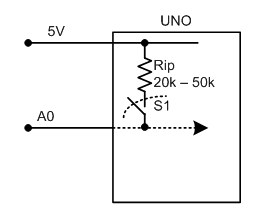# Counter implementation on IR sensor

Hello, I am currently working on a code for my versity project where two individual outputs fire at a time depending on different number of rotations. I am counting revolutions by IR sensor.I am facing troubles doing it. I am still getting outputs even if there's no input given on the IR. My code is below, can someone tell me what is wrong in it. TIA
(PS: I am kinda new in this so pardon me for any mistake )

``````int x = 0;
int cycle = 0;
int state = 0;

void setup() {
pinMode(A0, INPUT);
pinMode(A2, INPUT);
pinMode(9, OUTPUT);
pinMode(10, OUTPUT);
Serial.begin(9600);
}

void loop() {
if (state == 0) {
switch (x) {
case 1: state = 1; cycle = cycle + 1; break; //measuring the revolution
case 0: state = 0; break;
}
}

if (x == LOW) {
state = 0;
}

Serial.println(cycle);

if (cycle > 0) {
if (cycle % 2 != 0) { //odd numbers of revolution will give output in pin 9
digitalWrite(9, HIGH);
digitalWrite(10, LOW);
}
else if (digitalRead(A0) == 0) { //no input so no output
digitalWrite(9, LOW);
digitalWrite(10, LOW);
}
}

if (cycle % 2 == 0) {
if (digitalRead(A2) == 1) { //even numbers of rotation will fire pin 10
digitalWrite(9, LOW);
digitalWrite(10, HIGH);
}
else if (digitalRead(A2) == 0) {
digitalWrite(9, LOW);
digitalWrite(10, HIGH);
}
}
}

}
``````

we are missing a diagram describing how things (what things) are wired together.

your code is complicated to read because variables do not convey any meaning. what does it mean if x is HIGH or LOW ? (and what is x anyway). What does state 0 represent (you could use enum to get more meaningful names instead of 0 and 1 - or just use a bool if you have 2 states)

what's the use for

``````      case 0: state = 0; break;
``````

if you enter the if that leads to that switch/case, you know state is 0 already...

what is a revolution?

Depending on the sensor, you may need a pullup/pulldown resistor or some debouncing.

@saminyasar8936
1. Example of Input Line with Internal (built-in) Pull-up Resistor (Fig-1).Figure-1:

Code:

``````pinMode(A0, INPUT_PULLUP);
``````

2. Example Input Line without Internal Pull-up Resistor but with externally installed pull-up Resistor (Fig-2).Figure-2:

Code:

``````pinMode(A0, INPUT);
``````

3. Example of Input Line with Externally installed Pull-down Resistor (Fig-3).Figure-3:

Code:

``````pinMode(A0, INPUT);
``````

This topic was automatically closed 120 days after the last reply. New replies are no longer allowed.